AP Chemistry : Molecules

Example Questions

2 Next →

Example Question #5 : Other Molecular Concepts

A compound is comprised only of carbon and hydrogen. The molar mass of the compound is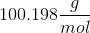and the compound is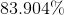carbon by mass. What is the empirical formula?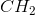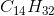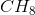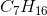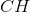Explanation:

Use the molar mass and percent by mass of carbon to find the moles of carbon in the compound.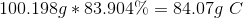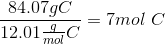We know that the compound is made solely of carbon and hydrogen. This allows us to find the percent by mass of hydrogen.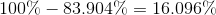Use the molar mass and the percent by mass of hydrogen to find moles of hydrogen in the compound.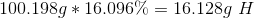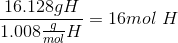The molecular formula is.

Because 7 and 16 have no common factors, the compound cannot be further reduced (in a numerical, not chemical sense).is also the empirical formula.

Example Question #6 : Other Molecular Concepts

A compound is comprised of sulfur and element X. The compound has a molar mass of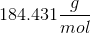and is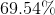sulfur. Analysis shows the compound has twice as much sulfur as element X. Find the molecular and empirical formulas for the compound, including the identity of element X.

Molecular: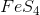Empirical: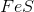Molecular: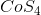Empirical:Molecular: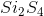Empirical: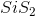Molecular:Empirical:Molecular:Empirical:Molecular:Empirical:Explanation:

Use the molar mass and percent by mass of sulfur to find the moles of sulfur in the compound.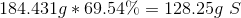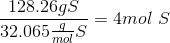Since the compound consists of only sulfur and element X, we can subtract to find the percent by mass of element X.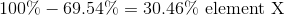Use the percent by mass to find the mass per mole of compound of element X.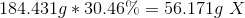Because we are told there are one-half as many moles of X as there are of sulfur, we know there are 2 moles of X (as compared to the 4 moles of sulfur). Divide the mass of element X by the moles of element X to find its atomic mass and its identity.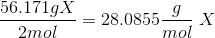By consulting a periodic table, we can find that element X is silicon.

Therefore, the molecular formula isand the empirical formula is.

Example Question #7 : Other Molecular Concepts

A compound is comprised of sodium and element X. The compound has a molar mass of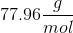and is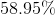sodium by mass. The compound consists of a 1:1 ratio between sodium and element X. Find the molecular and empirical formulas of the compound, including the identity of element X.

Molecular: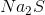Empirical: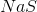None of these are correct

Molecular: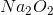Empirical: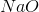Molecular:Empirical:Molecular:Empirical:Molecular:Empirical:Explanation:

Use the molar mass and percent by mass of sodium to find the moles of sodium in the compound.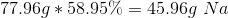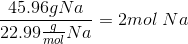Since the compound consists of only sodium and element X, we can subtract to find the percent by mass of element X.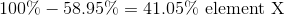Use the percent by mass to find the mass per mole of compound of element X.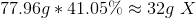Because there is a 1:1 ratio, between sodium and element X, they must have an equal number of moles in the compound. Since there are 2 moles of sodium, there must also be 2 moles of element X. Divide the mass of element X by the moles of element X to find its atomic mass and its identity.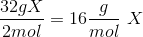By consulting a periodic table, we can find that element X is oxygen.

Therefore element X is Oxygen.

Therefore, the molecular formula isand the empirical formula is.

2 Next →